Courses

# Previous year Questions (2016-20) - Chemical Kinetics & Nuclear Chemistry Notes | EduRev

## JEE : Previous year Questions (2016-20) - Chemical Kinetics & Nuclear Chemistry Notes | EduRev

The document Previous year Questions (2016-20) - Chemical Kinetics & Nuclear Chemistry Notes | EduRev is a part of the JEE Course Chemistry Class 12.
All you need of JEE at this link: JEE

Q.1. During the nuclear explosion, one of the products is 90Sr with half-life of 6.93 years. If 1 µg of 90Sr was absorbed in the bones of a newly bom baby in place of Ca, how much time, in years, is required to reduce it by 90% if it is not lost metabolically?    (2020)
Ans. (23.03)

Given, half-life of 90Sr = 6.93 year; amount = 1 × 10–6g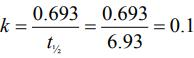From the first order kinetic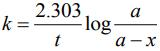For 90% decay of 90Sr,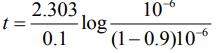⇒ t = 23.03 log 10
⇒ t = 23.03 years

Q.2. For the reaction
2H2(g) + 2NO(g) → N2(g) + 2H2O(g),
the observed rate expression is, rate =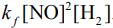. The rate expression for the reverse reaction is    (2020)

(1) kb[N2][H2O]2
(2)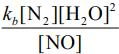(3) kb [N2][H2O]
(4)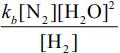Ans. (4)

Given, rate expression for reaction,
2H2 (g) + 2NO(g) → N2(g) + 2H2O(g)

rf=kf[NO]2[H2]

Now,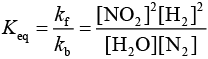Rearranging the above equation,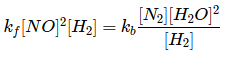=> rf = rb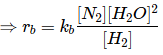Q.3. The rate of a certain biochemical reaction at physiological temperature (T) occurs 10times faster with enzyme than without it. The change in the activation energy upon adding enzyme is    (2020)
(1) −6(2.303)RT
(2) −6RT
(3) +6(2.303)RT
(4) +6RT
Ans. (1)

From Arrhenius equation without enzyme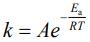(1)
With enzyme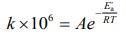(2)
On Dividing Eq. (1) by (2), we get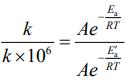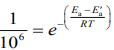(2)
Taking log of Eq. (3), we get
E'a - E= - 6(2.303)RT

Q.4.Consider the following plots of rate constant versus 1/T for four different reactions. Which of the following orders is correct for the activation energies of these reactions?    (2020)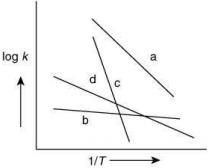(1) Eb > Ea > Ed > Ec
(2) Ea > Ec > Ed > Eb
(3) Ec > Ea > Ed > Eb
(4) Eb > Ed > Ec > E
a
Ans. (3)

From Arrhenius equation without enzyme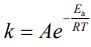Taking log of Eq. (1), we get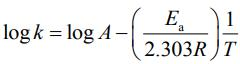By plotting log k against 1/T,  the slope =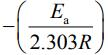and intercept = log A
From the graph of four different reaction,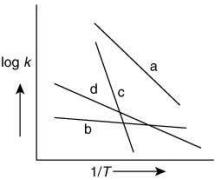The plot having high value of slop will have high value of activation energy. Thus, the correct order for activation energy of the reactions is: Ec > Ea > Ed > Eb.

Q.5. For the following reactions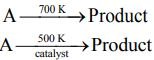it was found that the Ea is decreased by 30 kJ mol–1 in the presence of catalyst. If the rate remains unchanged, the activation energy for catalyzed reaction is (Assume pre exponential factor is same)    (2020)
(1) 75 kJ mol–1
(2) 105 kJ mol–1
(3) 135 kJ mol–1
(4) 198 kJ mol–1
Ans. (1)

Given, Eal = Ea and Ea2 = Ea - 30
T1 = 700 K and T2 = 500 K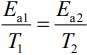(1) [Given, Rate remains unchanged]
Substituting the values in Eq. (1), we get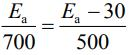⇒ 500Ea = 700Ea - 2100
⇒ 2100 = 700Ea - 500Ea
⇒ 2100 = 200Ea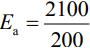⇒ Ea = 105 KJ mol-1
Thus, activation energy in presence of catalyst is Ea2 = E- 30
⇒ Ea2 = 105 - 30 = 75 kJ mol-1

Q.6. A sample of milk splits after 60 min. at 300 K and after 40 min. at 400 K when the population of lactobacillus acidophilus in it doubles. The activation energy (in kJ mol−1) for this process is closest to ________.    (2020)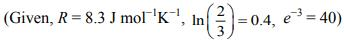Ans. (3.98)

Using Arrhenius equation at two different temperature,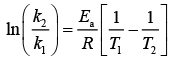(1)
Since, milk splits after 60 min. at 300 K and after 40 min. at 400 K.
Substituting the values in Eq. (1), we get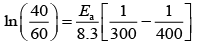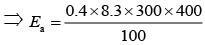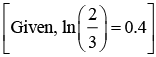⇒ Ea = 3.984 kJ mol−1

Q.7. The following results were obtained during kinetic studies of the reaction;
2A + B → Products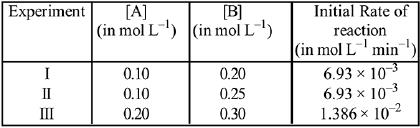The time (in minutes) required to consume half of A is:    (2019)
(1) 5
(2) 10
(3) 1
(4) 100
Ans.
(1)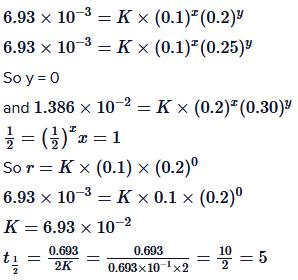Q.8. For the reaction, 2A + B → products, when the concentrations of A and B both were doubled, the rate of the reaction increased from 0.3 mol L-1s-1 to 2.4 mol L-1s-1. When the concentration of A alone is doubled, the rate increased from 0.3 L-1s-1 to 0.6 mol L-1s-1. Which one of the following statements is correct?    (2019)
(1) Total order of the reaction is 4
(2) Order of the  reaction with respect to B is 2
(3) Order of the reaction with respect to B is 1
(4) Order of the reaction with respect to A is 2
Ans.
(2)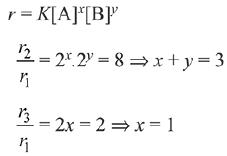∴ y = 2

Q.9. Consider the given plots for a reaction obeying Arrhenius equation (0°C < T < 300°C): (K and Ea are rate constant and activation energy, respectively)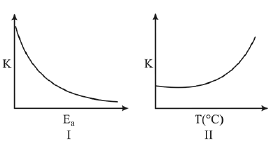Choose the correct option:    (2019)
(1) I is right but II is wrong
(2) Both I and II are correct
(3) I is wrong but II is right
(4) Both I and II are wrong
Ans.
(2)
From Arrhenius equation,
K= Ae-Ea/RT
So, as Ea increases, e-Ea/RT decreases, K decreases and as T increases, Ea/RT decreases, e-Ea/RT increases,

Q.10. For an elementary chemical reaction,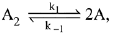the expression for d[A]/dt is:    (2019)
(1) k1[A2] - k-1[A]2
(2) 2k1[A2] - k-1[A]2
(3) k1 [A2] + k-1[A]2
(4) 2k1[A2] - 2k-1[A]2
Ans.
(4)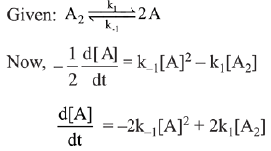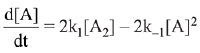Q.11. If a reaction follows the Arrhenius equation, the plot lnk vs 1/(RT) gives straight line with a gradient (-y) unit. The energy required to activate the reactant is:    (2019)
(1) y/R unit
(2) y unit
(3) yR unit
(4) - y unit
Ans.
(2)
From Arrhenius equation,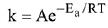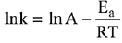slope = -y (given)
-y = -Ea
⇒  Ea = y

Q.12. The reaction 2X → B is a zeroth order reaction. If the initial concentration of X is 0.2 M, the half-life is 6 h. When the initial concentration of X is 0.5 M, the time required to reach its final concentration of 0.2 M will be:    (2019)
(1) 9.0 h
(2) 12.0 h
(3) 18.0 h
(4) 7.2 h
Ans.
(3)
For zero order reaction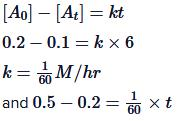t=18 hrs

Q.13. Decomposition of X exhibits a rate constant of 0.05  μg/year. How many years are required for the decomposition of 5 μg of X into 2.5  μg?    (2019)
(1) 50
(2) 25
(3) 20
(4) 40
Ans.
(1)
Rate constant of decomposition of X= 0.05 mg/year. Unit of rate constant confirms that the decomposition of X is a zero order reaction.
For zero order kinetics,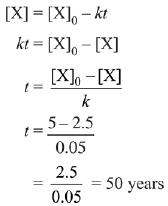Q.14. For a reaction, consider the plot of In k versus 1/T given in the figure. If the rate constant of this reaction at 400 K is 10-5 s-1, then the rate constant at 500 K is:    (2019)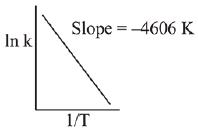(1) 10-6 s-1
(2) 2 x 10-4 s-1
(3) 10-4 s-1
(4) 4 x 10-4 s-2
Ans.
(3)
From Arrhenius equation,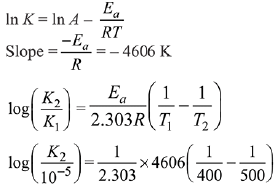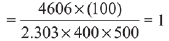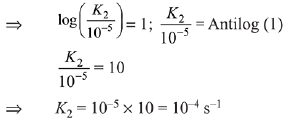Q.15. For the reaction 2A + B → C, the values of initial rate at different reactant concentrations are given in the table below.
The rate law for the reaction is:    (2019)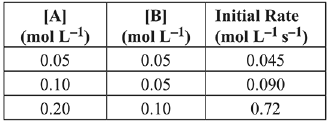(1) Rate = k[A][B]2
(2) Rate = k[A]2[B]2
(3) Rate = k[A][B]
(4) Rate = k[A]2[B]
Ans.
(1)
2A + B → C
Rate = k[A]x [B]y
Exp-1, 0.045 = k[0.05]x [0.05]y    ...(i)
Exp-2, 0.090 = k[0.1]x [0.05]y    ...(ii)
Exp-3, 0.72 = k[0.2]x [0.1]y    ...(iii)
Divide equation (i) by equation (ii)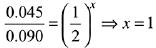Divide equation (i) by equation (iii)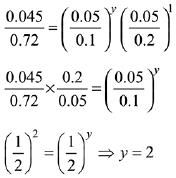Rate law = k[A]1 [B]2.

Q.16. For a reaction scheme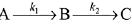if the rate of formation of B is set to be zero then the concentration of B is given by:    (2019)
(1) (k1 - k2) [A]
(2) k1k2 [A]
(3) (k1 + k2) [A]
(4) (k1/k2) [A]
Ans.
(4)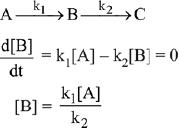Q.17. The given plots represents the variation of the concentration of a reactant R with time for two different reactions (i) and (ii). The respective orders of the reactions are:    (2019)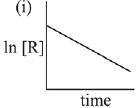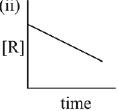(1) 1, 0
(2) 1, 1
(3) 0, 1
(4) 0, 2
Ans.
(1)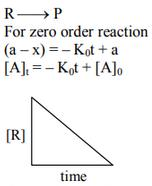For First order reaction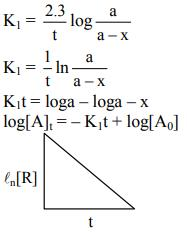Q.18. A bacterial infection in an internal wound grows as N2(t) = N0 exp(t), where the time t is in hours. A dose of antibiotic, taken orally, needs 1 hour to reach the wound. Once it reaches there, the bacterial population goes down as dN/dt = -5N2. What will be the plot of N0/N vs. t after 1 hour?    (2019)
(1)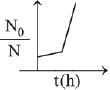(2)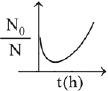(3)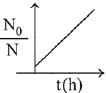(4)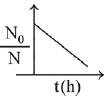Ans.
(3)
When drug is administered bacterial growth is given by dN/dt = -5N2
On integrating the above equation,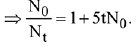The above equation is similar to straight line equation with positive slope.
Thus N0/Nt increases linearly with t.

Q.19. For the reaction of H2 with I2, the rate constant is 2.5 x 10-4 dm3 mol-1 s-1 at 327°C and 1.0 dm3 mol-1 s-1 at 527°C. The activation energy for the reaction, in kJ mol-1 is: (R = 8.314J K-1 mol-1)    (2019)
(1) 166
(2) 150
(3) 72
(4) 59
Ans.
(1)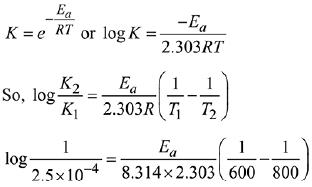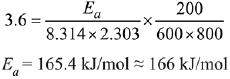Q.20. In the following reaction: xA → yB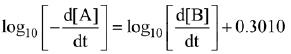‘A' and ‘B’ respectively can be:    (2019)
(1) n-Butane and Iso-butane
(2) C2H2 and C6H6
(3) C2H4 and C4H8

(4) N2O4 and NO2
Ans.
(3)
xA → yB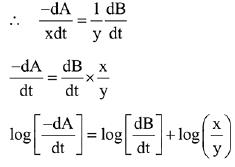Comparing this equation with the equation given in question. We get,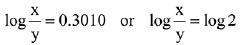∴ x/y = 2
∴ The reaction is of type 2A → B.
Hence, option (3) is correct.

Q.21. NO, required for a reaction is produced by the decomposition of N2O5 in CCl4 as per the equation,
2N2O5(g) → 4NO2(g) + O2(g).

The initial concentration of N2O5 is 3.00 mol L-1 and it is 2.75 mol L-1 after 30 minutes. The rate of formation of NO2 is:    (2019)
(1) 4.167 x 10-3 mol L-1 min-1
(2) 1.667 x 10-2 mol L-1 min-1
(3) 8.333 x 10-3 mol L-1 min-1
(4) 2.083 x 10-3 mol L-1 min-1
Ans.
(2)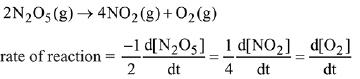According to the question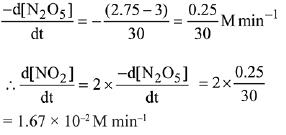Q.22. At 5180C, the rate of decomposition of a sample of gaseous acetaldehyde, initially at a pressure of 363 Torr, was 1.00 Torr s-1 when 5% had reacted and 0.5 Torr s-1 when 33% had reacted. The order of the reaction is:    (2018)
(1) 2
(2) 3
(3) 1
(4) 0
Ans. (1)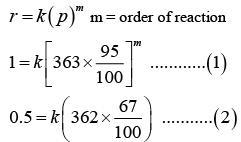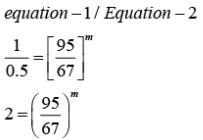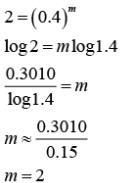Q.23. N2O5 decomposes to NO2 and O2 and follows first order kinetics. After 50 minutes, the pressure inside the vessel increases from 50 mm Hg to 87.5 mm Hg. The pressure of the gaseous mixture after 100 minute at constant temperature will be:    (2018)
(1) 106.25 mm Hg
(2) 116.25 mm Hg
(3) 136.25 mm Hg
(4) 175.0 mm Hg
Ans. (1)
2N2O5 → 4NO2 + O2
p − 2x         4x         x
pt = p − 2x + 4x + x
pt = p + 3x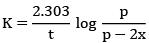at  t = 0,   pt = p = 50 mm Hg
at  t = 50 mm,   pt = 87.5 mm Hg
p + 3x = 87.5
p = 87.5 − 3x
50 = 87.5 − 3x
12.5 = x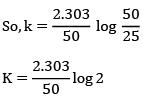p − 2x = 50 − 2(12.5) = 25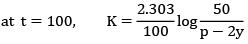Since K will remain same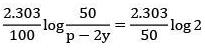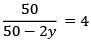50 = 50 × 4 − 8y
50 = 200 − 8y
8y = 150
y =  18.75
pt = p + 3y
= 50 + 3 (18.73) = 106.25 mm Hg

Q.24. If 50 % of a reaction occurs in 100 second and 75 % of the reaction occurs in 200 second, the order of this reaction is:    (2018)
(1) 2
(2) 3
(3) Zero
(4) 1
Ans. (4)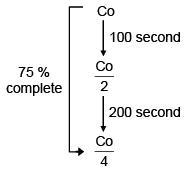First order reaction as half life is constant.

Q.25. Two reactions R1 and R2 have identical pre - exponential factors. Activation energy of Rexceeds that of Rby 10 kJ mol–1. If k1 and k2 are rate constants for reactions R1 and R2 respectively at 300 K, then ln (k1/ k2) is equal to
(R = 8.314 J mole–1 K–1)    (2017)
(1) 8
(2) 12
(3) 6
(4) 4
Ans.  (4)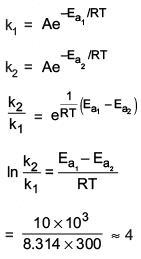Q.26. The rate of a reaction A doubles on increasing the temperature from 300 to 310 K. By how much, the temperature of reaction B should be increased from 300 K so that rate doubles if activation energy of the reaction B is twice to that of reaction A.    (2017)
(1) 4.92 K
(2) 9.84 K
(3) 19.67 K
(4) 2.45 K
Ans.
(1)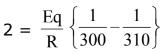...(i)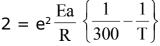...(ii)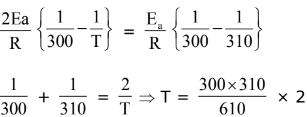= 304.92

Q.27. The rate of a reaction quadruples when the temperature changes from 300 to 310 K. The activation energy of this reaction is:
(Assume activation energy and pre-exponential factor are independent of temperature; ln 2 = 0.693; R = 8.314 J mol-1 K-1)    (2017)
(1) 53.6 kJ mol-1
(2) 214.4 kJ mol-1
(3) 107.2 kJ mol-1
(4) 26.8 kJ mol-1
Ans.
(3)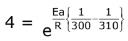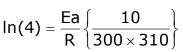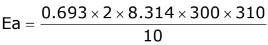= 107165.79 J = 107.165 KJ

Q.28. Decomposition of H2O2 follows a first order reaction. In fifty minutes the concentration of H2O2 decreases from 0.5 to 0.125 M in one such decomposition. When the concentration of H2O2 reaches 0.05 M, the rate of formation of O2 will be:    (2016)
(1) 6.93 x 10-2 mol min-1
(2) 6.93 x 10-4 mol min-1
(3) 2.66 L min-1 at STP
(4) 1.34 x 10-2 mol min-1
Ans. (2)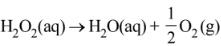For a first order reaction
K = 2.303/t log a/(a-x)
Given a = 0.5, (a-x) = 0.125, t = 50 min
∴ k = 2.303/50 log 0.5/0.125
= 2.78 x 10-2 min-1
r = d[H2O2] = 2.78 x 10-2 x 0.05
= 1.386 x 10-3 mol min-1
Now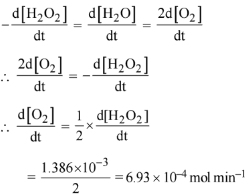Q.29. The reaction of ozone with oxygen atoms in the presence of chlorine atoms can occur by a two step process show below:
O3(g) + Cl*(g) → O2(g) + ClO*(g) ...(i)
ki = 5.2 × 109 L mol-1 s-1
ClO*(g) + O*(g) → O2(g) + Cl*(g) ...(ii)
[Kii = 2.6 x 1010 L mol-1s-1]
The closest rate constant for the overall reaction O3(g) + O*(g) → 2O2(g) is:    (2016)
(1) 1.4 × 1020 L mol-1 s-1
(2) 5.2 × 109 L mol-1 s-1
(3) 3.1 × 1010 L mol-s-1
(4) 2.6 × 1010 L mol-1 s-1
Ans.
(2)
The rate constant of overall reaction depends slowest step. Hence equation(i) is slowest step. Option(2) is correct.

Q.30. The rate law for the reaction below is given by the expression k [A][B]
A + B → Product
If the concentration of B is increased from 0.1 to 0.3 mole, keeping the value of A at 0.1 mole, the rate constant will be:    (2016)
(1) 9 k
(2) 3 k
(3) k/3
(4) k
Ans.
(4)
Rate constant is independent of concentration.

Offer running on EduRev: Apply code STAYHOME200 to get INR 200 off on our premium plan EduRev Infinity!

,

,

,

,

,

,

,

,

,

,

,

,

,

,

,

,

,

,

,

,

,

;